Home >> Class 9 >>

# Atoms & molecules Class 9 Science Chapter, Explanation, Notes, Question Answers

## CBSE Class 9 Science Chapter 3 Atoms & molecules, Explanation, Examples, Question Answers

### Chapter 3 - Atoms & molecules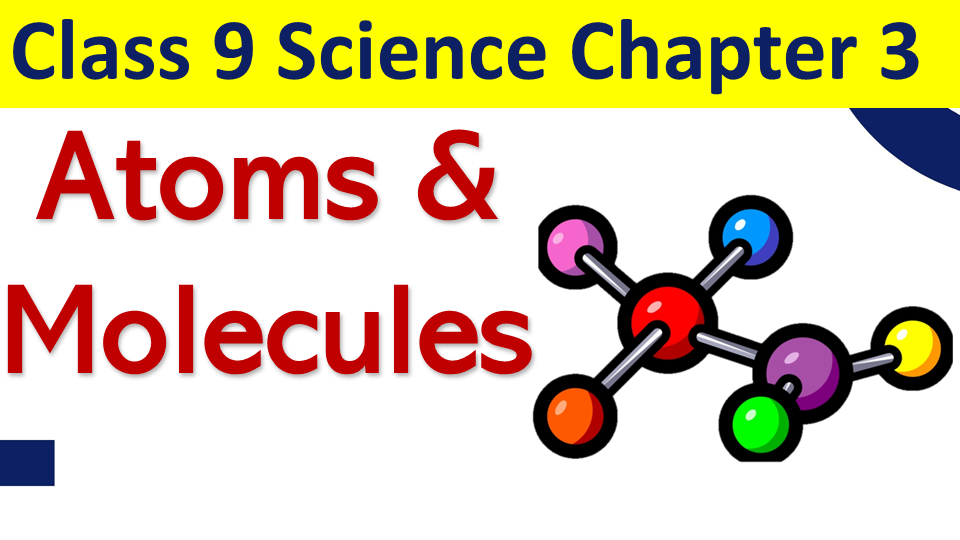Topics to be covered

We come across different things around us like chair, table, etc. and all the things that surround us have mass and weight. They all constitute matter. Matter is anything that occupies space and has mass. Matter is made up of small particles called atoms.

### Dalton's atomic theory

According to Dalton?s Atomic Theory,

• All matter is made up of small particles called atoms.
• Atom is invisible.
• Atom is indivisible.
• Atoms of an element are alike in all aspects.
• Atoms of different elements combine in fixed whole number ratios to form compounds.

(f) Atoms can neither be created nor destroyed.

Drawbacks of Dalton?s Atomic Theory were as follows-

1. According to Dalton, an atom is indivisible but later on it was proved that atom can be subdivided into electrons, protons and neutrons.
2. Atoms of an element can somehow differ from each other.

So, these drawbacks led to the failure of Dalton?s theory of an atom.

We also know that a lot of chemical reactions take place in our day to day life like making of tea, changing milk to curd, making cheese from milk and lots more. All the chemical reactions taking place obey certain set of laws. Let us study these chemical reactions and the rules that they obey.

Chemical reaction

The process by which some substances react to form a new substance. The substances which react are called reactants and the new ones formed are called products.

For example: A+B🡪C+D
In this, A and B are reactants and C and D are called products.
For example: when we make tea, we add sugar, tea, milk and water. We mix them and heat the mixture. The result is that we get a new substance that is tea.

### Laws of chemical combination

There are certain sets of laws that are obeyed by all chemical reactions. These laws are given by Lavoisier & Joseph. Let us study them in detail.

Law of conservation of mass
According to this law, ?matter can not be created nor destroyed in a chemical reaction.? That is, it always remains constant.
Like, in all chemical reactions the total mass of products is equal to the total mass of reactants.

For example:-
2H2 + O2 🡪 2 H2O
(Reactants) (Product)

=> 4 + 2 x 16.32 🡪 2 x 18
=> 36 g?? 🡪? 36 g.
This example shows that the total mass of reactants is equal to the total mass of products formed which is in accordance with the law.
Law of constant proportions: (Law of definite proportion)
According to it, in a chemical substance, the same elements are always present in a definite proportion by mass and volume irrespective of the method of preparation involved.

For example, In Co2 molecule, the elements that form it remain the same that is carbon and oxygen and also, the ratio of C and O remains the same that is 3:8 by mass (i.e. 12.32) and 1 : 2 by volume.

### Atom

It is the smallest particle of an element which may as may not have an independent existence. An atom is very small in size. The size is measured in unit of nm (nanometer).
1nm ? 10-9 m.
Hydrogen atom is the smallest of all. Its size is only 10-10 nm.
Atoms are represented by symbols (given by Berzelius).

### IUPAC and Atomic symbols

IUPAC - International Union Of Pure & Applied Chemistry
The IUPAC approves the names of elements.
According to it,

1. Symbol if an element is either the first letter of the name of the element or the first two letters of the name of an element.
2. In case there are two letters, then the first letter is in a capital case and the second letter is in small case.

For example- lead is written as ?Pb? and not as ?pb?.
Sometimes, the symbol is derived from the Latin name of an element.
For example, if we look at the symbol of Sodium- ?Na? and not ?So? because it is derived from its Latin name that is ?Natrium?. Similarly, for Potassium, Copper, Iron, Mercury, etc. Latin names and symbols of a few elements are given below: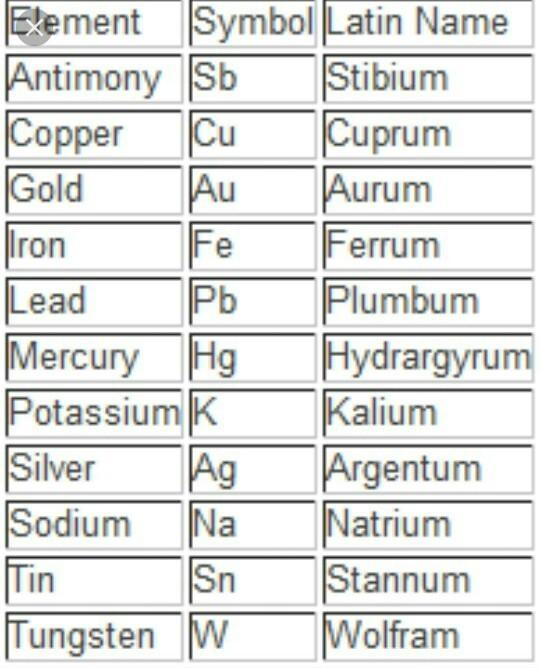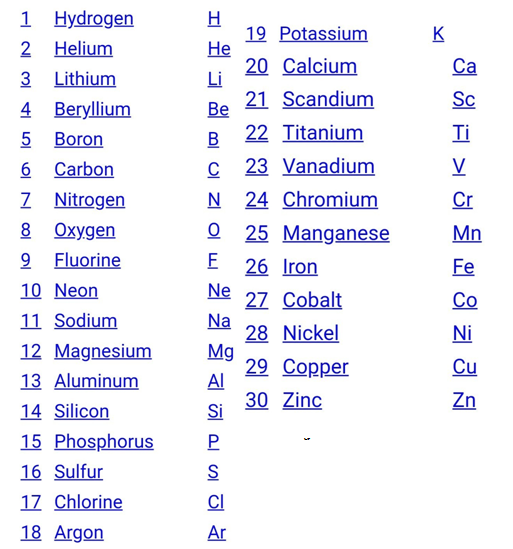### Atomic mass of element

If we try to find the exact mass of an atom, we can?t find it. The reason being that an atom is too small in size that it is difficult to measure its size and moreover, the size of an atom changes, depending upon its neighbouring? atoms. Therefore, instead of finding its absolute mass, we try to find the relative mass of an atom. The standard element chosen for finding relative mass is carbon-6.
The relative atomic mass of an atom of an element is defined as the average relative mass of an atom as compared to an atom of 612c taken as 12u.

Atomic mass: In simple language, atomic mass is the number of times an atom of an element is heavier than 1/12th of a Carbon atom.
For example: If we say that the atomic mass of Sodium is 23, it means that sodium is 23 times heavier than 1/12th of a carbon atom.

### Molecule

The particle next to an atom is a molecule, but it has an independent existence and is formed when atoms combine with each other. Let us study about it.
A Molecule is the smallest particle of an element or compound which is able to exist independently.
For example, carbon dioxide molecule, hydrogen gas molecule, etc .
But it has been seen that some molecules are formed by the combination of two atoms of the same kind like H2 and some may have two or more atoms of different kinds like H2O. This shows that in a molecule any number of atoms can be present and they may be of the same or different kinds.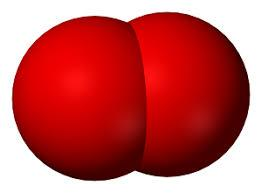Atomicity: It is the number or the kinds of atoms present in a molecule of an element.
Some more examples:
Phosphorous exists as 🡪 P4 (tetra atomic).
Sulphur exists as 🡪 S8 (polyatomic).
Nitrogen exists as 🡪 N2 (diatomic).

### Compound

When atoms of two or more different elements combine together in a definite proportion by mass and volume, it forms a compound.
for example:- H 2O is formed only when the ratio of H and O is 2:1 by volume and ratio by mass is 1:8.

### Ion

We know that the atom is neutral, that is, the number of positive and negative charges in it is equal but if sometimes, an atom gains or loses negative or positive charge, it no longer remains neutral. It becomes an ?ion?. Let us study about it.

Ion:? The atom that bears a charge is called an ?ion?.
Ion is of two types:
1. Cation
2. Anion

Cation: they are positively charged ions. They are formed when an atom loses a negative charge. Therefore, they always have negative charges less than normal atoms.

Example: Na ?1 negative charge 🡪Na+ (cation)
Anion: they are negatively charged ions and are formed when an atom gains a negative charge. Therefore, they have negative charges more than normal atoms.
Example: S +2 negative charges 🡪 S2- (anion)

The group of atoms that bears a charge is also called a polyatomic ion. The following radicals are given below: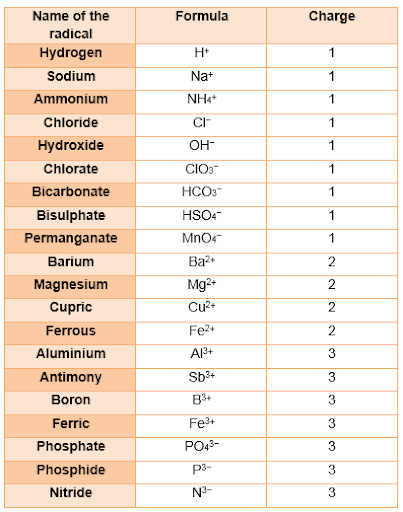### Chemical formula

Chemical Formula is the symbolic representation of compound.
For example, the compound ammonium nitrate is written as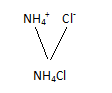If we say that water is represented as H2O, we get the following information from it-

1. H2O represents water.
2. H2O represents one molecule of water.
3. It represents that 1molecule of H2O contain 2Hydrogen and 1 Oxygen atoms.
4. It represents 18g of water.
5. It represents that 1 molar mass of water has 6.022 x 10 23 molecules.

### Mass percentage

Mass Percentage is the ratio of mass of a substance whose percentage is to be calculated to the total mass of the substance multiplied by 100.

### Mole Concept

Mole Concept is equal to 6.023 x 10 entities present in a substance. It is also called Avagadro?s number.
Mole = given mass of substance to its atomic or molecular mass.

### NCERT book solutions

Q1. In a reaction, 5.3 g of sodium carbonate reacted with 6 g of ethanoic acid. The products were 2.2 g of carbon dioxide, 0.9 g water and 8.2 g of sodium ethanoate. Show that these observations are in agreement with the law of conservation of mass.

Sodium carbonate + ethanoic acid ? sodium ethanoate + Carbon dioxide +water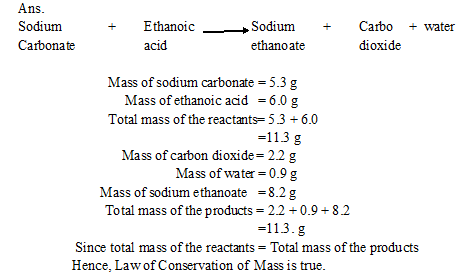Q2. Hydrogen and oxygen combine in the raito of 1 : 8 by mass to form water. What mass of oxygen gas would be required to react completely with 3 g of hydrogen?

Ans. One g of hydrogen gas reacts with oxygen = 8 g
3 g of hydrogen gas will react with oxygen = 3?8 = 24 g

Q3. Which postulate of Dalton?s atomic theory is the result of the law of conservation of mass?

Ans. Law of conservation of mass is based upon the postulate that atoms are indivisible particles and can neither be created nor destroyed during any chemical reaction.

Q4. Which Postulate of Dalton?s atomic theory can explain the law of definite proportions?

Ans. Atoms of various elements combine in simple whole numbers but fixed ratio to form compound atoms (molecules).

Q5. Define the atomic mass unit.
Ans. One atomic mass unit is a mass unit and it is equal toth of the mass of an atom of carbon-12.
1 a.m.u. = 1.66 ? 10-27 ?kg.

Q6. Why is it not possible to see and atom with naked eyes?

Ans: It is not possible to see and atom with naked eyes because atoms are very very small. The radius of an atom is of the order of 10-10 m.

Q7.Write down the formulae of
(1) Sodium oxide
(2) Aluminum chloride
(3) Sodium Sulphide
(4) Magnesium hydroxide

Ans.

• Sodium oxide- Na2O
• Aluminium chloride- AlCl3
• Sodium sulphide- Na2S
• Magnesium Hydroxide- Mg(OH)2

Q.8 Write down the name of the compounds represented by the following formulae
(1) AI2 (SO4)3????????????
(2) CaCl2???????????????????
(3) K2SO4
(4) KNO3???????????????????????????????
(5) CaCO3

Ans.
(1) AI2 (SO4)3 - Aluminum sulphate?????????
(2) CaCl2 - Calcium Chloride
(3) K2SO4 - Potassium sulphate???
(4) KNO3?? Potassium nitrate??????????
(5) CaCO3 Calcium carbonate

Q9. What is meant by the term chemical formula?

Ans. Chemical Formula - The chemical formula of a substance indicates its constituent elements and number of atoms of each combining element present in one molecule of such sunstance.

Q10. How many atoms are present in a
(1) H2S molecule ????????????????
(2) Po43- ion?
Ans. (1) An H2 S molecule represents the following-
(a) Two atoms of hydrogen and ??????????????
(b)One atom of sulphur.

(2) Po43- ??represents the following-
(a) One atom of? P???????????????????????????????????????
(b) three atoms of? O.

Q11. Calculate the molecular mass of?? H2, O2, l2, CO2, CH2,C2H6, C2H4, NH3,CH3OH.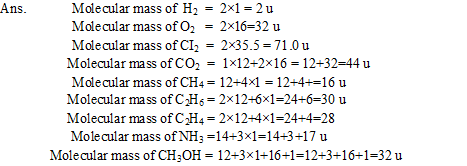Q12. Calculate the formula unit masses of ZnO, Na2O, K2CO3, given atomic masses of Zn = 65 u, Na = 23 u, C =12u, and O = 16u.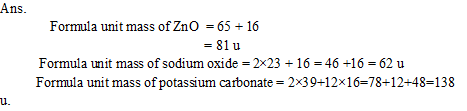13. If one mole of carbon atoms weighs 12 gram, what is the mass (in grams) of 1atom of carbon?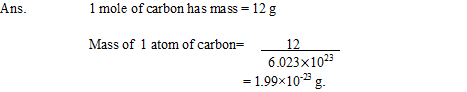Q14. Which has more number of atoms, 100 grams of sodium or 100 grams of? Iron (given, atomic mass of? Na =23 u, Fe =56 u ) ?Q15. A 0.24 g sample of a compound of oxygen and boron was found by analysis to contain 0.096 g of boron and 0.144 g of oxygen. Calculate the percentage composition of the compound by weight.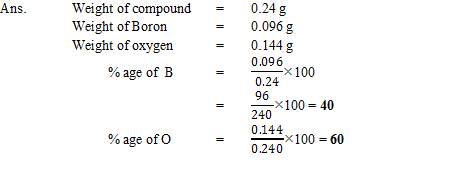Q16.? When 3.0 g of carbon is burnt in 8.00 g oxygen, 11.00 g of carbon dioxide is produced. What mass of carbon dioxide will be formed when 3.00 g of carbon is burnt in 50.00 g of oxygen ? Which law of chemical combination will govern your answer?

Ans. 3.00 g of carbon combines with 8.00 g of oxygen to produce 11.00? g of carbon dioxide

C + O2 = CO2 ----->

When 3.00 g of carbon is burnt in 50.00 g of oxygen, 11.00 g of carbon dioxide is produced. This is based upon the law of constant composition.

Q17. What are polyatomic ions ? Give examples.

Ans. Polyatomic ions: A group of atoms carrying a charge (+ve or-ve) is called a polyatomic ion.
Example SO 24 - SO 23 - NH + 4 etc.

Q18.Write the chemical formulae of the following
(a) Magnesium chloride
(b) Calcium oxide
(c) Copper nitrate
(d) Aluminium chloride
(e) Calcium carbonate

Ans.
(a) Magnesium chloride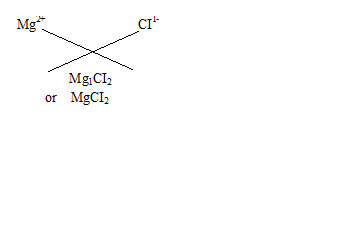(b) Calcium oxide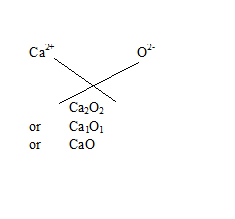(c) Copper nitrate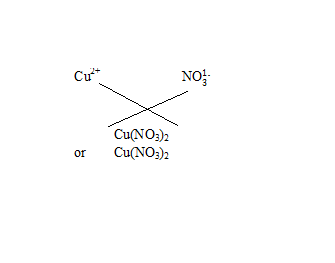(d)Aluminium chloride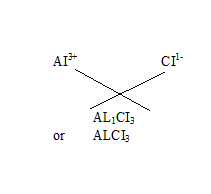(e)? Calcium carbonate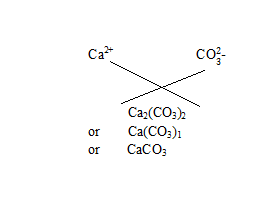Q19. Give the names of the elements present in the following compounds
(a) Quick lime
(b) Hydrogen bromide
(c) Baking powder
(d) Potassium sulphate.

Ans.
(a) Quick lime (CaO). The elements present in it are calcium and oxygen.
(b) Hydrogen? bromide (HBr). The elements present in it are hydrogen and bromine.
(c) Baking powder (NaHCO3). The elements present in it are sodium, hydrogen, carbon and oxygen.
(d) Potassium sulphate (K2SO4). The elements present in it are potassium, sulphur and oxygen.

Q20. Calculate the molar mass of the following substances
(a) Ethyne, C2H2
(b) Sulphur molecule, S8
(c) Phosphorus molecule, P4 (Atomic mass of phosphors is 31)
(d) Hydrochloric acid, HCI
(e) Nitric acid, HNO3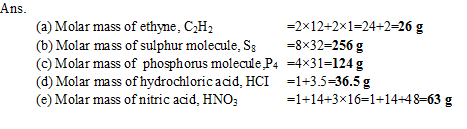Q21.What is mass of
(a) 1 mole of nitrogen atom
(b) 4 moles of Aluminum atoms (Atomic mass of Aluminum = 27 ?
(c) 10 moles of Sodium sulphite (Na2SO3)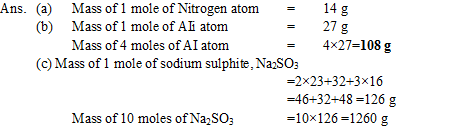Q22. Convert into mole:
(a) 12g of oxygen gas
(b) 20g of water
(c) 22g of carbon dioxide

Ans: (a) In Oxygen gas: moles = 12/32= 0.375
(b) In water: moles =20/18=1.11
(c) In carbon dioxide =22/44=0.5

Q23. What is the mass of: (a) 0.2 mole of oxygen atoms? (b) 0.5 mole of water molecules?

A.
(a) Mass = mole Atomic Mass
= 2/10 16
= 32/10 = 3.2g
(b) Mass = mole Atomic Mass
0.5 = mass 18
mass = o.5/18?10
= 1/36 = 0.02g

Q24. Calculate the number of molecules of sulphur (S8) present in 16 g of solid sulphur.

A. Number of Molecules = mole Avogadro Number
= given mass / M mass Avogadro Number
= 16/256 6.023?10
= 0.0625 6.023 10
= 0.376 10
= 3.7 10

Q25. Calculate number of Aluminum ions present in 0.051g of aluminum oxide?

Ans: Mass of 1 mole of aluminum ions = 27g
Number of moles in 0.056g of aluminum ions ? = 0.051/27
Number of aluminum ions = 0.051/27 x 6.022 x 1023
=1.178 x 1021